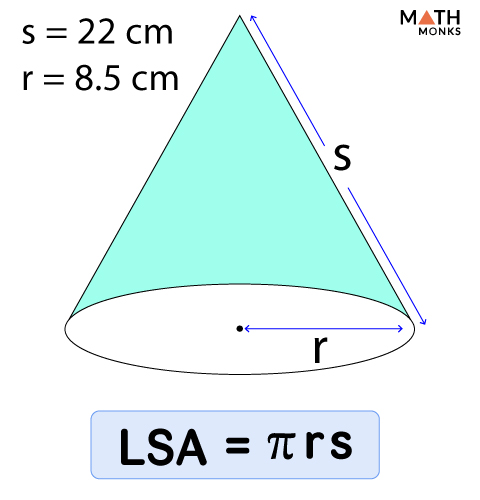# Surface Area of a Cone

The surface area (total surface area) of a cone is the entire space occupied by the flat circular base of the cone and its curved surface. The surface area is expressed in square units such as m2, cm2, mm2, and in2. Here we will discuss how to find the surface area of a right circular cone.

## Formula

The basic formula when slant height and radius are known is:

### Derivation

Let us consider a cone with radius r, circumference c, and slant height s.

So, C = 2πr,

And Base Area (B) of the cone = πr2

Now, we unroll the lateral face of the cone first. When placed flat, the lateral face becomes a sector of a circle as shown below. Circumference c becomes the arc length, and s becomes the radius of the sector.

As we know,

Area of a sector (A) ${=\dfrac{1}{2}cs}$, where C = arc length, s = radius,

= ½ × 2πr × s, [∵ C = 2πr]

= πrs = Lateral Surface Area (LSA) of a cone

Here we have derived the formula of LSA.

Now, Surface Area (SA) = LSA + B

∴ SA = LSA + B

= πrs + πr2

## Solved Examples

Find the surface area of a cone with a radius of 7 cm and a slant height of 15 cm.

Solution:

As we know,
Surface Area (SA) = πr2 + πrs, here r = 7 cm, s = 15 cm, π = 3.141
∴ SA = 3.141 × 72 + 3.141 × 7 × 15
= 483.71 cm2Find the area of the curved surface of a cone with a radius of 8.5 cm and a slant height of 22 cm.

Solution:

As we know,
Lateral Surface Area (LSA) = πrs, here r = 8.5 cm, s = 22 cm, π = 3.141
∴ LSA = 3.141 × 8.5 × 22
= 587.36 cm2